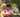### JavaScript assignment operators

February 10, 2020

JavaScript assignment operators

NameShorthand operatorMeaning
Assignmentx = yx = y
Addition assignmentx += yx = x + y
Subtraction assignmentx -= yx = x - y
Multiplication assignmentx *= yx = x * y
Division assignmentx /= yx = x / y
Remainder assignmentx %= yx = x % y
Exponentiation assignmentx **= yx = x ** y
Left shift assignmentx <<= yx = x << y
Right shift assignmentx >>= yx = x >> y
Unsigned right shift assignmentx >>>= yx = x >>> y
Bitwise AND assignmentx &= yx = x & y
Bitwise XOR assignmentx ^= yx = x ^ y
Bitwise OR assignmentx |= yx = x | yPosted by Tai Lu ( 呂台生 ) who's building useful things.You should follow him on Twitter